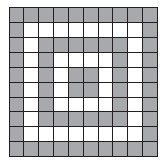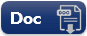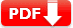# Percentages Practice Test SET 2

### Percentages Practice Test Question Answers

SET: 2

 1. Richard and Michael deposited $3000 and$4000 in a company at the rate of 10% per annum for 3 years and 2½ years respectively. The difference of the amounts received by them will be 🔘 A. $10 🔘 B.$100 🔘 C. $1000 🔘 D.$1100 🔘 E. $– 1000 Show Answers Answer: D 1100 (formula of Simple interest I = (P × R × T)/100)  2. If 90% of x is 315 km, then the value of x is 🔘 A. 3 km 🔘 B. 30 km 🔘 C. 300 km 🔘 D. 315 km 🔘 E. -3 km Show Answers Answer: D 315 KM. (90% of x is 315 km i.e (90/100) × x = 315)  3. The percent that represents the unshaded region in the figure.🔘 A. 40% 🔘 B. 50 🔘 C. 60% 🔘 D. 70% 🔘 E. 75% Show Answers Answer: A. 40% Total number of parts in the given figure = 10 × 10 = 100 and unshaded parts = (40/100) × 100  4. If the price of oil is decreased by 20% per barrel, then the new price of 3 barrel of oil originally costing$120 will be 🔘 A. $60 🔘 B.$82 🔘 C. $96 🔘 D.$100 🔘 E. $110 Show Answers Answer:$96 (new price of oil= 120 – 20% of original price therefore  120 – (20/100) × 120
 5. Benicio purchased a vintage car for $50,59,700 and spent he$40300 on its repairs. To make a profit of 5%, he should sell the car for $__? 🔘 A.$5155000 🔘 B. $5255000 🔘 C.$5355000 🔘 D. $5455000 🔘 E.$5555000
Answer: C . $5355000 (formula: Profit % = (profit/CP) × 100 )  6. Kylo bought 120 pens at$4 each. He sold 60 % of the pens at $5 each and the remaining at$3.50 each. If he made profit then find out his profit percentage. 🔘 A. 10% 🔘 B. 30% 🔘 C. 100% 🔘 D. 10.10% 🔘 E. 1%
Answer: A 10%  , Profit percentage = (Profit/CP) × 100
 7. Joseph bought a certain number of apples out of which 4% were bad. After selling  75% of the remaining he had 96 apples. Find the number of apples he bought. 🔘 A. 300 🔘 B. 400 🔘 C. 600 🔘 D. 800 🔘 E. 900
 Document Type Download Link Free Editable Doc FileFree Printable PDF File